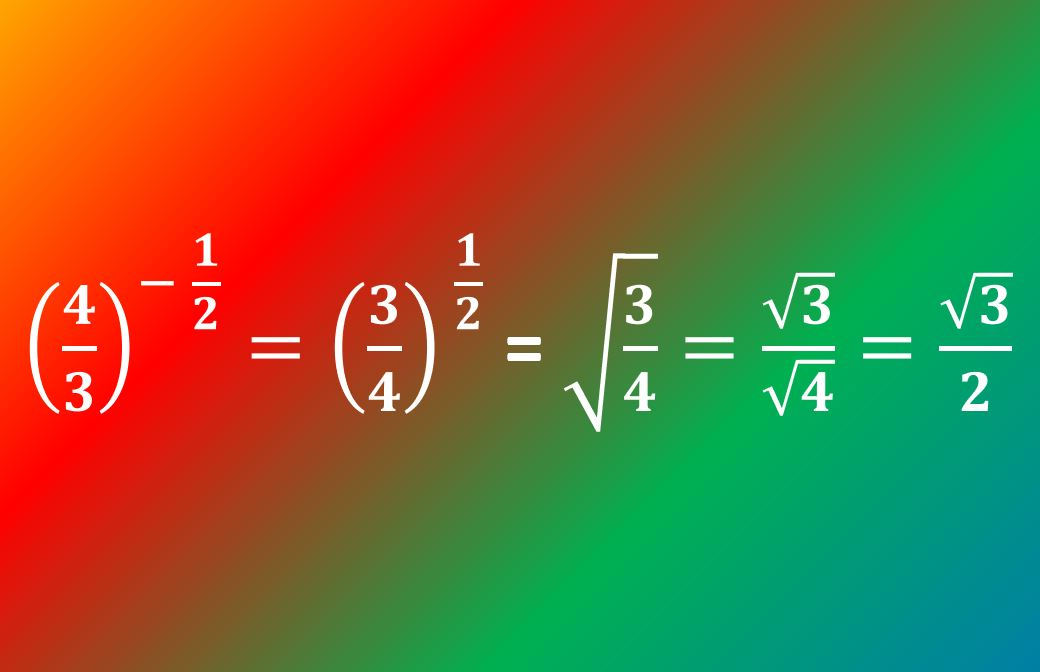# Fractional indices: Essential skills for GCSE and A-level Maths

Part 1 covers all the Foundation content – basics, laws of indices, negative indices – and you can find it here.

In this post we’re picking up where that one left off, and moving on to look at fractional indices (needed for Higher GCSE spec and for A-level).

The image below shows an example of the kind of expression using fractional indices that you can expect to encounter and should be able to handle.

## The laws of indices

We covered these in the previous lesson, but here’s a reminder:

• 1st law: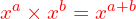• 2nd law: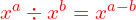• 3rd law: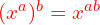## How do fractional indices work?

Take a look at the expressions below, which involve simple fractional indices, and apply the laws of indices to simplify them as far as possible:

1.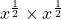2.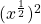3.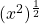In each case you should get the same answer:, which of course is simply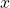.

What do you have to multiply by itself to get? Compare your answer with example 1.
What do you have to square to get? Compare your answer with example 2.
What do you have to do to get from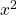to just? Compare your answer with example 3.

In each case we need the square root…

… so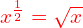What if we’d started with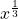? How many of those would you need to multiply together to get?

Answer: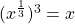… so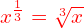And more generally,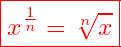Note: As with surds, a fractional power indicates only the positive root; you only need to worry about the possibility of a second, negative, root if you’ve started off with the statement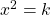or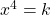, etc.

#### Your turn: Work out the values of:

1.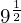2.3.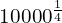4.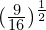5.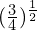## What if the numerator is greater than 1?

If the numerator is not simply 1 then we need to apply the 3rd law, but having the power in fractional form makes it look a little different from what we’ve encountered before: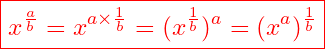Of course,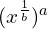is the same as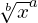and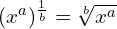; both give the same result so it doesn’t matter whether you do the root first and then the integer power, or the integer power and then the root – though generally it’s easiest to take the root first.

#### Now evaluate:

1.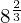2.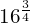3.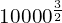4.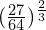5.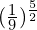This “flower power” meme might help you to remember how to deal with fractional indices:

Remember, any number to the power of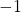is its own reciprocal. It doesn’t actually matter whether you do the reciprocal bit first or the fractional indices bit first, but I’d usually suggest getting rid of the negative powers as early as possible.

Here’s an example: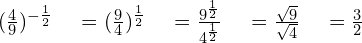Now here are some for you to have a go at:

1.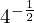2.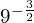3.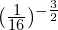4.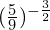5.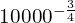6.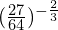7.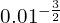And of course the example in the image at the beginning of this article should make sense to you now too!

## Mixing it up with algebra

Now have a go at these questions involving a mixture of negative and fractional indices combined with algebraic expressions. Some of them are quite challenging – take care to double-check your working as it’s very easy to make mistakes!

1. Express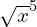in index form.
2. Expand and simplify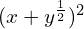, giving your answer in surd form (i.e. using surds rather than indices).
3. Given that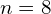, evaluate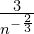4. Given that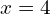, evaluate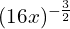.
5. Express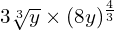in surd form.
6. Simplify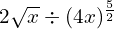.

If you got all of those right then you’ve done exceptionally well!

Here’s a great codebreaker activity from JustMaths that I use with my Higher Tier and A-level students to practise negative and fractional indices (it downloads as a PDF).

And that covers fractional indices!

I hope you’ve found this post helpful. If you have then please share it, and also please take a look at the rest of my site to see what other help I can offer.

### How do fractional indices work?

1.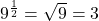2.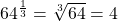3.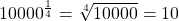4.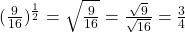5.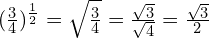(Yes, you will sometimes end up with surds!)

Back to this section

#### Numerator greater than 1

1.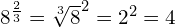2.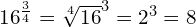3.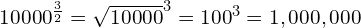4.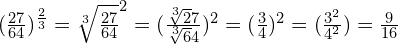5.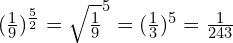Back to this section

1.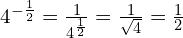2.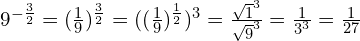3.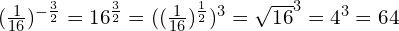4.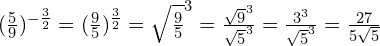5.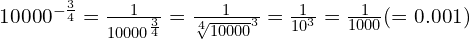6.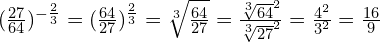7.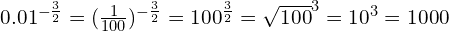#### Mixing it up with algebra

1.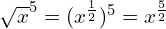2.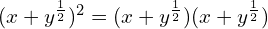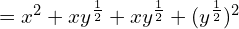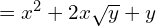3.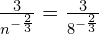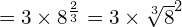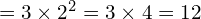4.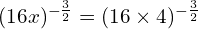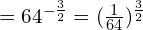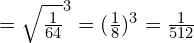5.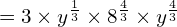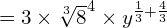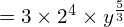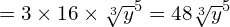6.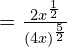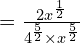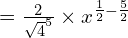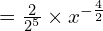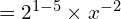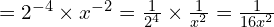.